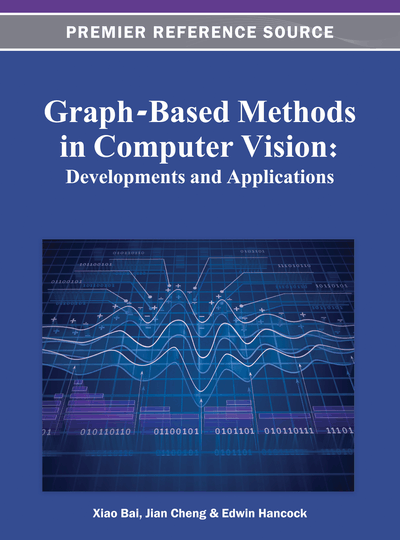# Hypergraph Based Visual Segmentation and Retrieval

Yuchi Huang (General Electric Global Research, USA)
DOI: 10.4018/978-1-4666-1891-6.ch006
Available
\$37.50
No Current Special Offers

## Abstract

This chapter explores original techniques for the construction of hypergraph models for computer vision applications. A hypergraph is a generalization of a pairwise simple graph, where an edge can connect any number of vertices. The expressive power of the hypergraph models places a special emphasis on the relationship among three or more objects, which has made hypergraphs better models of choice in a lot of problems. This is in sharp contrast with the more conventional graph representation of visual patterns where only pairwise connectivity between objects is described. This chapter draws up from three aspects to carry the discussion of hypergraph based methods in computer vision: (i) The advantage of the hypergraph neighborhood structure is analyzed. The author argues that the summarized local grouping information contained in hypergraphs causes an ‘averaging’ effect which is beneficial to the clustering problems; (ii)The chapter discusses how to build hypergraph incidence structures and how to solve the related unsupervised and semi-supervised problems for two different computer vision scenarios: video object segmentation and image retrieval; (iii) For the application of image retrieval, the chapter introduces a novel hypergraph model — probabilistic hypergraph to exploit the structure of the data manifold by considering not only the local grouping information, but also the similarities between vertices in hyperedges.
Chapter Preview
Top

## 1. Introduction

In computer vision and other applied machine learning problems, a fundamental task is to cluster a set of data in a manner such that elements of the same cluster are more similar to each other than elements in different clusters. In these problems, we generally assume pairwise relationships among the objects of our interest. For example, a common distance measure for data points lying in a vector space is the Euclidean distance, which is used in a lot of unsupervised central clustering methods such as k-means (Macqueen, 1967), k-centers clustering (Macqueen, 1967) and affinity propagation (Frey, 2007). Actually, a data set endowed with pairwise relationships can be naturally organized as a pairwise graph (for simplicity, we denote the pairwise graph as simple graph in the following). The simple graph partitioning problem in mathematics consists of dividing a graph G into k pieces, such that the pieces are of about the same size and there are few connections between the pieces. With a few notable exceptions, the similarities between objects in a simple graph are utilized to present the pairwise relationships. The simple graph can be undirected or directed, depending on whether the relationships among objects are symmetric or not. Usually a computed kernel matrix is associated to a directed or undirected graph, a lot of methods for unsupervised and semi-supervised learning can then be formulated in terms of operations on this simple graph and achieve better clustering results compared to central clustering methods (Shi, 2000) (Ng, 2001) (Luxburg,2008).

However, in many real world problems, it is not complete to represent the relations among a set of objects as simple graphs. This point of view is illustrated in a good example used in (Zhou, 2006). In this example, a collection of articles need to be grouped into different clusters by topics. The only information that we have is the authors of all the articles. One way to solve this problem is to construct an undirected graph in which two vertices are connected by an edge if they have at least one common author (Table 1 and Figure 1), and then spectral graph clustering can be applied (Fieldler, 1973) (Chan, 1993) (Shi, 2000). Each edge weight of this undirected graph may be assigned as the number of authors in common between two articles. It is an easy, nevertheless, not natural way to represent the relations between the articles because this graph construction loses the information whether the same person is the author of three or more articles or not. Such information loss is unexpected because the articles by the same person likely belong to the same topic and hence the information is useful for our grouping task. A more natural way to remedy the information is to represent the data points as a hypergraph. An edge in a hypergraph is called a hyperedge, which can connect more than two vertices; that is, a hyperedge is a subset of vertices. For this article clustering problem, it is quite straightforward to construct a hypergraph with the vertices representing the articles, and the hyperedges the authors (Figure 1). Each hyperedge contains all articles by its corresponding author. Furthermore, positive weights may be put on hyperedges to emphasize or weaken specific authors’ work. For those authors working on a smaller range of fields, we may assign a larger weight to the corresponding hyperedge. Compared to a simple pairwise graph, in this example hypergraph structure more completely illustrates the complex relationships among authors and articles.

Table 1.

## Complete Chapter List

Search this Book:
Reset